Next: Appendices Up: QED Processes Previous: Vertices

# Problems

1. Show that the plane-wave solutions normalized as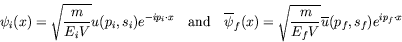have the desired Lorentz transformation properties. In particular, include the effect of a Lorentz transformation on the box volume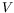to show that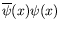is a scalar and that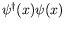is the time component of a vector, as desired.

2. Prove the following:
1.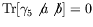,
2.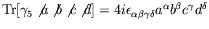,
3.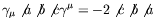,
4.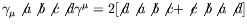,
5.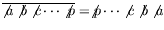.

3. Construct the amplitude for bremsstrahlung in electron-proton scattering and show that the static limit reduces to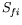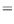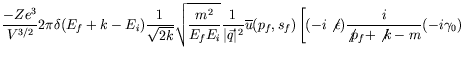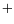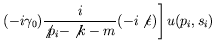(7.384)

for bremsstrahlung in a Coulomb field. Show that there is the same correspondence in factors between these two cases as was found in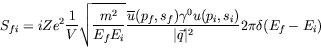and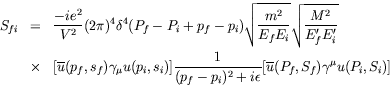for elastic scattering.

4. Derive the Bethe-Heitler cross-section for production of an electron-positron pair by an incident photon in a Coulomb field. Show that the amplitude for this is related to the bremsstrahlung amplitude in equation (7.385) by the substitution rule.

5. Calculate the cross-section for the absorption of light by a bound electron in an atom with low atomic number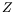, such that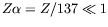and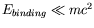. Assume also that the frequency of the light is such that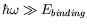. Making these simplifying assumptions calculate differential and total cross-sections for the two limiting cases:

1.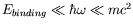non-relativistic ,
2.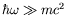ultra-relativistic .

6. Construct the differential cross-section for electron-electron scattering in the lowest order Born approximation in terms of laboratory energies and scattering angles.

7. Show that the scattering matrix (S-matrix) is unitary.

8. Derive the Bethe-Heitler cross-section for bremestrahlung of photons of arbitrary energy.

9. Verify that the inverse of the momentum space operator'' of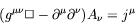does not exist.

10. In the center-of-mass frame for the process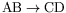show that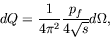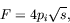and hence that the differential cross-section is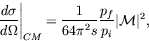where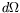is the element of solid angle about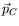,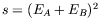,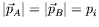, and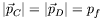.

11. Show that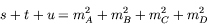where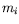is the rest mass of particle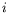.

12. Taking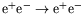to be the-channel process, verify that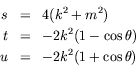where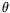is the center-of-mass scattering angle and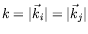, where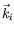and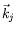are, respectively, the momenta of the incident and scattered electrons in the center-of-mass frame. Show that the process is physically allowed provided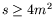,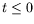, and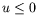. Note that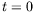(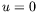) corresponds to the forward (backward) scattering

13. To show your understanding of QED, develope spinless QED. Calculate
1. compton scattering of bosons and
2. electro-production of pion pairs.

14. Rosenbluth's Formula. The realistic description of the scattering of an electron at a spin-1/2 hardon has to take into account the internal structure and anomalous magnetic moment of the hardon. To that end one replaces the transition current in momentum space which orignates from the Dirac equation with the more general bilinear expression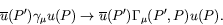(7.385)

1. Show that the most general expression for a transition current that fulfills the condition of Lorentz covariance, hermiticity, and gauge invariance can be written as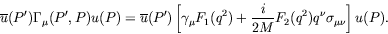(7.386)

Here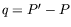is the momentum transfer and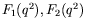are unspecified real functions (form factors'').

2. What is the physical meaning of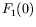and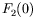? This can be deduced by studying the interaction energy with static EM fields in the nonrelativistic limit.

3. Calculate the unpolarized cross-section of electron scattering at a hardon with vertex function above in the ultrarelativistic limit.

15. Mandelstam variables applied to Møller and Bhabha scattering.
1. Show that the kinematics of any binary scattering process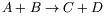can be expressed in terms of the three Lorentz-invariant Mandelstam variables.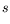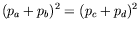(7.387)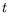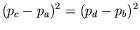(7.388)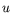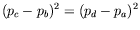(7.389)

Prove the identity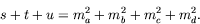(7.390)

2. Derive the differential cross-section for electron-electron and electron-positron scattering in terms of the Mandelstam variables. Do not neglect the electron mass in this calculation.

3. Write down explicit results for the Møller and Bhabha cross-sections in the centre-of-mass system and in the laboratory system.

16. Degree of polarization. Calculate the differential scattering cross-section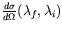for Coulomb scattering of electrons with longitudinal polarization. Derive the degree of polarization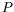as a function of the scattering angle.

17. Derive the differential and total unpolarized cross-sectiion for pair annhilation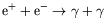in the centre-of-mass frame.

18. Derive the total unpolarized cross-section for creation of an electron-positron pair by two colliding photons,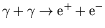. Express the result in terms of the velocity of the produced particles in the centre-of-mass frame. Hint: use the result for pair annhilation cross-section.

19. Calculate the cross-section of electron-positron pair creation by an incoming photon in the field of a heavy nucleus with charge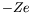. Hint: the calculation can be considereably simplified by exploting crossing symmetry which relates pair creation and bremsstrahlung.

20. Show that the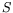-matrix is symmetric under charge conjugation.

21. We derived the laboratory frame cross-section for scattering of a high energy electron from an ideal'' (structureless) proton. However, the proton matrix element of the electromagnetic current was assumed to have the naive form. In reality one should use the current matrix element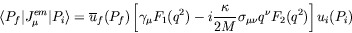where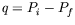is the momentum transfer,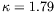is the anomalous magnetic moment of the proton, and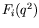are form factors which account for the finite hadronic size.

1. Calculate the high energy laboratory cross-section for electron-proton scattering using the full current element and show that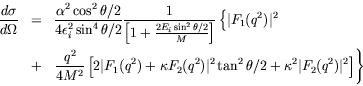where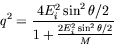2. Verify that this expression reduces to that given earlier in the limit that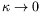and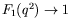.

This is the Rosenbluth cross-section which has been used in order to map out nuclear and particle charge distributions via electron scattering.

22. Suppose in our formalism we replace the vacuum by a Fermi gas with Fermi momentum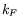. How is the Feynman propagator modified? Compute the change in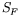in the low-density limit.

23. Show that the rate for an electron in the hydrogen-atom ground state to radiate and fall into empty negative-energy states (treated in Born approximation) in the energy interval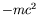to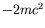is approximately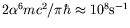.Next: Appendices Up: QED Processes Previous: Vertices
Douglas M. Gingrich (gingrich@ ualberta.ca)
2004-03-18